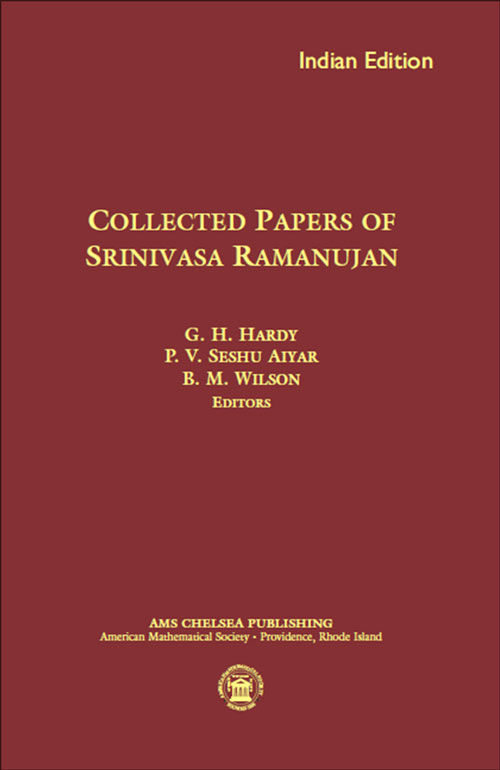Collected Papers of Srinivasa Ramanujan
G. H. Hardy, P. V. Seshu Aiyar, and B. M. Wilson
Price
1545.00
ISBN
9781470409180
Language
English
Pages
464
Format
Paperback
Dimensions
158 x 240 mm
Year of Publishing
2013
Territorial Rights
Restricted
Imprint
Universities Press
Catalogues

The influence of Ramanujan on number theory is without parallel in mathematics. His papers, problems, and letters have spawned a remarkable number of later results by many different mathematicians. Here, his 37 published papers, most of his first two and last letters to Hardy, the famous 58 problems submitted to the Journal of the Indian Mathematical Society, and the commentary of the original editors (Hardy, Seshu Aiyar and Wilson) are reprinted again, after having been unavailable for some time.

In this printing of Ramanujan''s collected papers, Bruce Berndt provides an annotated guide to Ramanujan''s work and to the mathematics it inspired over the last three-quarters of a century. The historical development of ideas is traced in the commentary and by citations to the copious references. The editor has done the mathematical world a tremendous service that few others would be qualified to do.

G. H. Hardy, P. V. Seshu Aiyar, and B. M. Wilson

• Some properties of Bernoulli's numbers
• On Question 330 of Prof. Sanjana
• Note on a set of simultaneous equations
• Irregular numbers
• Squaring the circle
• Modular equations and approximations to ?
• On the integral  ? x0tan-1t/t d t
• On the number of divisors of a number
• On the sum of the square roots of the first  n natural numbers
• On the product ? n=8n=0[1+(x/a+nd)3]
• Some definite integrals
• Some definite integrals connected with Gauss's sums
• Summation of a certain series
• New expressions for Riemann's functions  ? (s) and  ?(t)
• Highly composite numbers
• On certain infinite series
• Some formulæ in the analytic theory of numbers
• On certain arithmetical functions
• A series for Euler's constant ?
• On the expression of a number in the form  ax2+by2+cz2+du2
• On certain trigonometrical sums and their applications in the theory of numbers
• Some definite integrals
• Some definite integrals
• A proof of Bertrand's postulate
• Some properties of  p(n), the number of partitions of n
• Proof of certain identities in combinatory analysis
• A class of definite integrals
• Congruence properties of partitions
• Algebraic relations between certain infinite products
• Congruence properties of partitions
• Une formule asymptotique pour le nombre des partitions de n
• Proof that almost all numbers  n are composed of about  log log n prime factors
• Asymptotic formulæ in combinatory analysis
• Asymptotic formulæ for the distribution of integers of various types
• The normal number of prime factors of a number n
• Asymptotic formulæ in combinatory analysis
• On the coefficients in the expansions of certain modular functions
• Questions and solutions
• Appendix I: Notes on the papers
• Appendix II: Further extracts from Ramanujan's letters to G. H. Hardy
• Commentary on Ramanujan's collected papers
THE BOOKPOINT (INDIA) PVT. LTD.